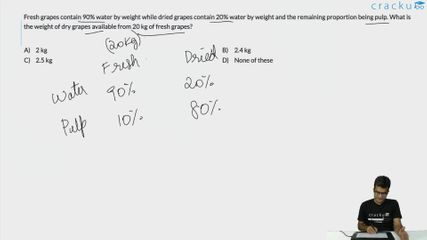Question 35

# Fresh grapes contain 90% water by weight while dried grapes contain 20% water by weight and the remaining proportion being pulp. What is the weight of dry grapes available from 20 kg of fresh grapes?

Solution

Fresh grapes contain 90% water so water in 20kg of fresh pulp = (90/100)x20= 18kg.

In 20kg fresh grapes, the weight of water is 18kg and the weight of pulp is 2kg.

The concept that we apply in this question is that the weight of pulp will remain the same in both dry and fresh grapes. If this grape is dried, the water content will change but pulp content will remain the same.

Suppose the weight of the dry grapes be D.

80% of the weight of dry grapes = weight of the pulp = 2 kg

(80/100) x D =2 kg.

D = 2.5 kg

### View Video Solution